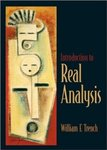# Introduction to Real Analysis

(0 reviews)

No ratings

William F. Trench, Trinity University

Publisher: A.T. Still University

Language: English

## Conditions of UseAttribution-NonCommercial-ShareAlike
CC BY-NC-SA

• Chapter 1: The Real Numbers
• Chapter 2: Differential Calculus of Functions of One Variable
• Chapter 3: Integral Calculus of Functions of One Variable
• Chapter 4: Infinite Sequences and Series
• Chapter 5: Structure of Rn
• Chapter 6: Vector-Valued Functions of Several Variables
• Chapter 7: Integrals of Functions of Several Variables
• Chapter 8: Metric Spaces

## Ancillary Material

• A.T. Still University

This is a text for a two-term course in introductory real analysis for junior or senior mathematics majors and science students with a serious interest in mathematics. Prospective educators or mathematically gifted high school students can also benefit from the mathematical maturity that can be gained from an introductory real analysis course.

The book is designed to fill the gaps left in the development of calculus as it is usually presented in an elementary course, and to provide the background required for insight into more advanced courses in pure and applied mathematics. The standard elementary calculus sequence is the only specific prerequisite for Chapters 1–5, which deal with real-valued functions. (However, other analysis oriented courses, such as elementary differential equation, also provide useful preparatory experience.) Chapters 6 and 7 require a working knowledge of determinants, matrices and linear transformations, typically available from a first course in linear algebra. Chapter 8 is accessible after completion of Chapters 1–5.